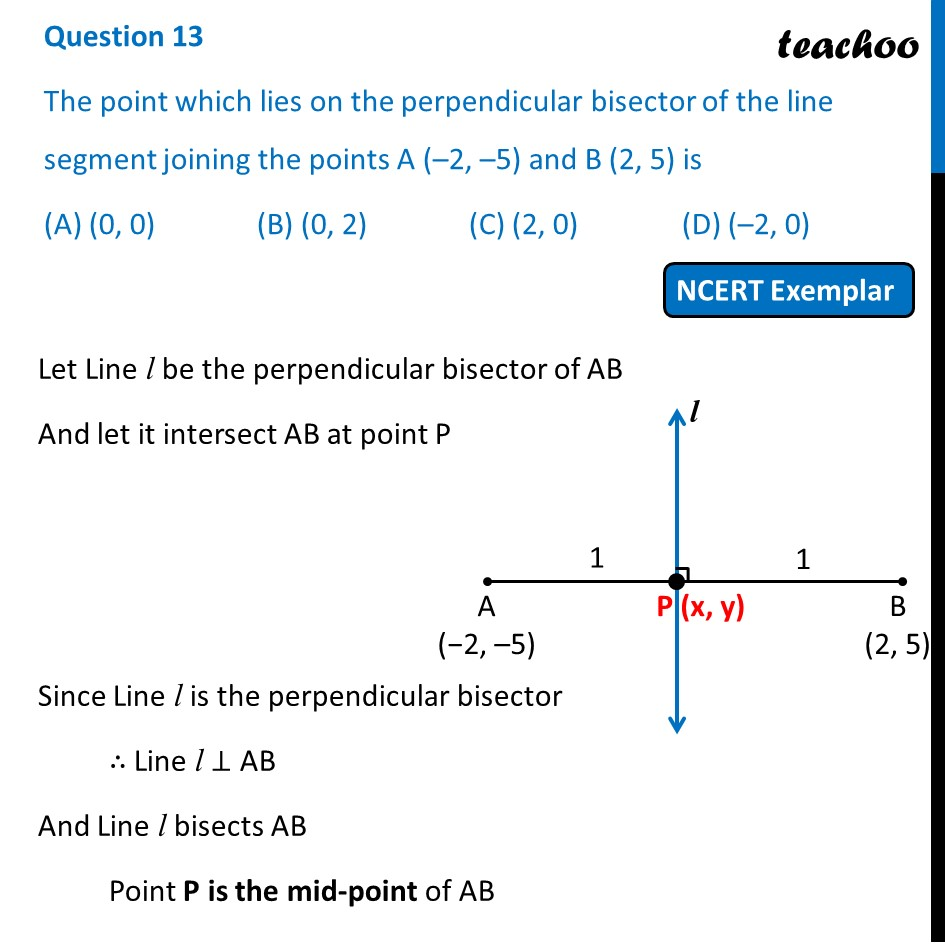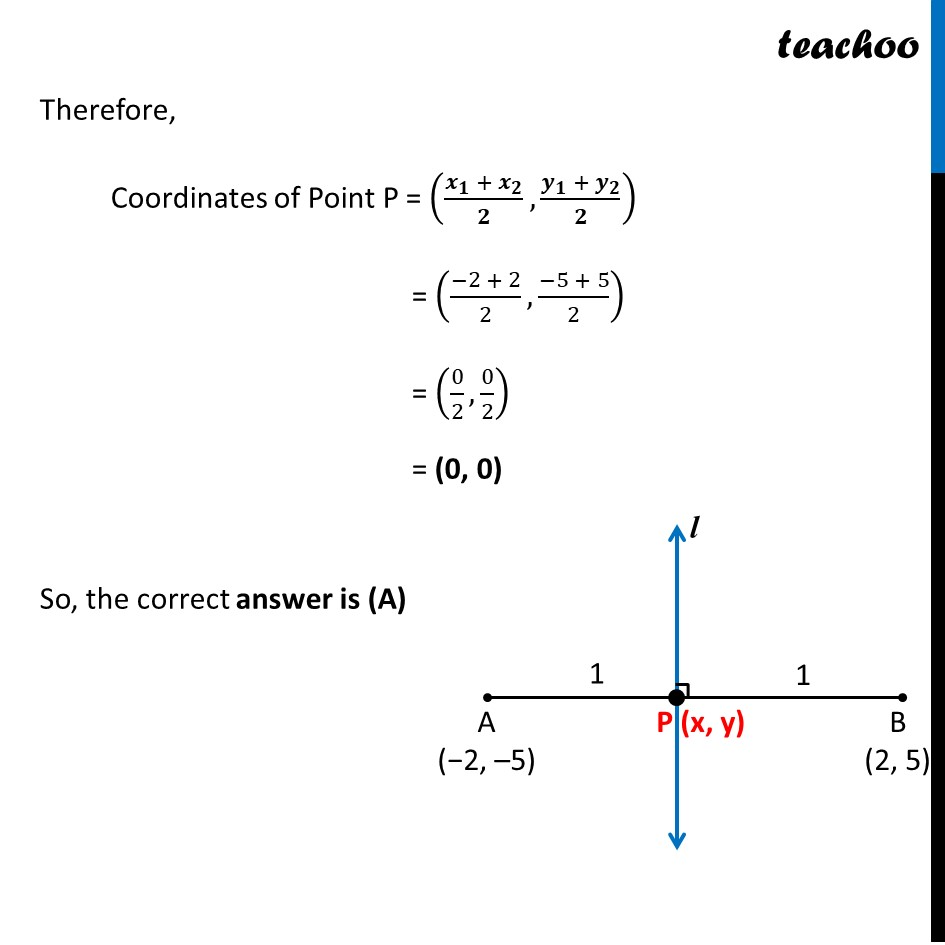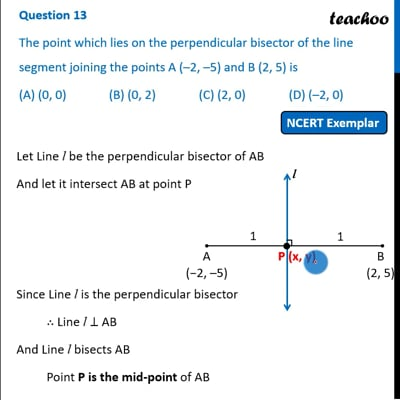NCERT Exemplar - MCQ

Chapter 7 Class 10 Coordinate Geometry
Serial order wise

## (A) (0, 0)   (B) (0, 2)   (C) (2, 0)   (D) (–2, 0)This video is only available for Teachoo black users

Learn in your speed, with individual attention - Teachoo Maths 1-on-1 Class

### Transcript

Question 13 The point which lies on the perpendicular bisector of the line segment joining the points A (–2, –5) and B (2, 5) is (A) (0, 0) (B) (0, 2) (C) (2, 0) (D) (–2, 0) Let Line l be the perpendicular bisector of AB And let it intersect AB at point P Since Line l is the perpendicular bisector ∴ Line l ⊥ AB And Line l bisects AB Point P is the mid-point of AB Therefore, Coordinates of Point P = ((𝒙_𝟏 + 𝒙_𝟐)/𝟐,(𝒚_𝟏 + 𝒚_𝟐)/𝟐) = ((−2 + 2)/2,(−5 + 5)/2) = (0/2,0/2) = (0, 0) So, the correct answer is (A)